# Chapter 4.2. More Complex Conditions – Exam Problems

The previous chapter introduced you to nested conditions in JavaScript. Via nested conditions, the program logic in a particular application can be represented using if conditional statements that are nested one into another. We also explained the switch-case conditional statement that allows selecting from a list of options. Now we are going to solve some practical exercises and make sure we have an in-depth understanding of the material, by discussing a set of more complex problems that had been given to students on exams. Before moving to the problems, let's first recall what nested conditions are:

## Nested Conditions

if (condition1) {
if (condition2)
// body;
else
// body;
}Remember that it is not a good practice to write deeply nested conditional statements (with more than three levels of nesting). Avoid nesting of more than three conditional statements inside one another. This complicates the code and makes its reading and understanding difficult.

## Switch-Case Conditions

When the program operation depends on the value of a variable, instead of doing consecutive checks with multiple if-else blocks, we can use the switch-case conditional statement.

switch (selector) {
case value1:
statement;
break;
case value2:
statement;
break;
default:
statement;
break;
}


The structure consists of:

• Selector - an expression that calculates a particular value.
• Multiple case labels followed by commands, ending in a break.

## Exam Problems

Now, after we refreshed our knowledge on how to use and nested conditional statements to implement more complex conditions and program logic, let's solve some exam problems.

## Problem: On Time for The Exam

A student has to attend an exam at a particular time (for example at 9:30 am). They arrive in the exam room at a particular time of arrival (for example 9:40 am). It is considered that the student has arrived on time if they have arrived at the time when the exam starts or up to half an hour earlier. If the student has arrived more than 30 minutes earlier, the student has come too early. If they have arrived after the time when the exam starts, they are late.

Write a program that inputs the exam starting time and the time of student's arrival, and prints if the student has arrived on time, if they have arrived early or if they are late, as well as how many hours or minutes the student is early or late.

### Input Data

Read the following four integers (arguments):

• The first line (argument) contains the exam starting time (hours) – an integer from 0 to 23.
• The second line (argument) contains the exam starting time (minutes) – an integer from 0 to 59.
• The third line (argument) contains the hour of arrival – an integer from 0 to 23.
• The fourth line (argument) contains minutes of arrival – an integer from 0 to 59.

### Output Data

Print the following on the first line on the console:

• "Late", if the student arrives later compared to the exam starting time.
• "On time", if the student arrives exactly at the exam starting time or up to 30 minutes earlier.
• "Early", if the student arrives more than 30 minutes before the exam starting time.

If the student arrives with more than one minute difference compared to the exam starting time, print on the next line:

• "mm minutes before the start" for arriving less than an hour earlier.
• "hh:mm hours before the start" for arriving 1 hour or earlier. Always print minutes using 2 digits, for example "1:05".
• "mm minutes after the start" for arriving more than an hour late.
• "hh:mm hours after the start" for arriving late with 1 hour or more. Always print minutes using 2 digits, for example "1:03".

### Sample Input and Output

Input Output Input Output
9
30
9
50
Late
20 minutes after the start
16
00
15
00
Early
1:00 hours before the start
9
00
8
30
On time
30 minutes before the start
9
00
10
30
Late
1:30 hours after the start
14
00
13
55
On time
5 minutes before the start
11
30
8
12
Early
3:18 hours before the start
Input Output
10
00
10
00
On time
11
30
10
55
Early
35 minutes before the start
11
30
12
29
Late
59 minutes after the start

### Hints and GuidelinesIt is recommended to read the assignment a few times, take notes and sketch the examples while thinking before you start with the code.

#### Processing The Input Data

According to the assignment, we expect four lines containing different integers to be passed. Examining the provided parameters, we can use the Number type, as it is suitable for the expected values. We simultaneously read the input data and parse the string value to the selected data type for the integer.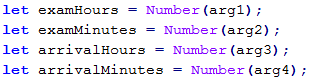Examining the expected output, we can create variables that contain the different output data types, to avoid using the so-called "magic strings" in the code.#### Calculations

After reading the input data, we can now start writing the logic for calculating the result. Let's first calculate the start time of the exam in minutes for easier and more accurate comparison:Let's also calculate the student arrival time using the same logic: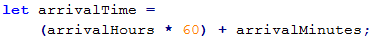What remains is to calculate the difference between the two times, to determine when and what time compared to the exam time the student arrived at:Our next step is to do the required checks and calculations, and finally, we will print the output. Let's separate the code into two parts.

• First, let's show when the student arrived – were they early, late or on time. To do that, we will use an if-else statement.
• After that, we will show the time difference, if the student arrives at a different time compared to the exam starting time.

To spare one additional check (else), we can, by default, assume that the student was late.

After that, according to the condition, we will check whether the difference in times is more than 30 minutes. If this is true, we assume that the student is early. If we do not match the first condition, we need to check if the difference is less than or equal to zero (<= 0), by which we are checking the condition whether the student arrived within the range of 0 to 30 minutes before the exam.

In all other cases, we assume that the student was late, which we set as default, and no additional check is needed: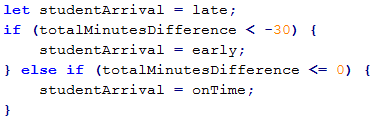Finally, we need to understand and print what is the time difference between exam start time and student arrival time, as well as whether this time difference indicates the time of arrival before or after the exam start.

We check whether the time difference is more than one hour, to print hours and minutes in the required format, or less than one hour, to print only minutes as a format and description.

We also need to do one more check – whether the time of student's arrival is before or after the exam start time.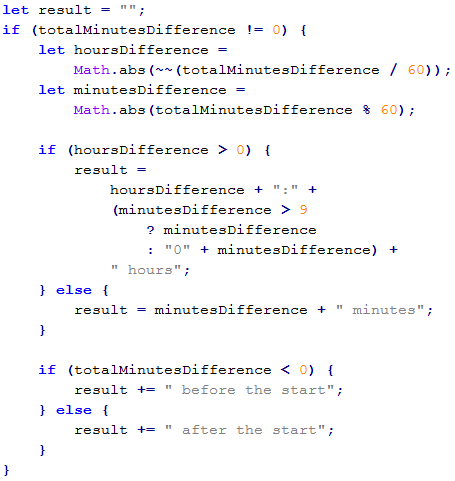#### Printing The Result

Finally, what remains is to print the result on the console. According to the requirements, if the student arrived right on time (not even a minute difference), we do not need to print a second result. This is why we apply the following condition: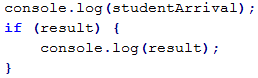Actually, for the task, printing the result on the console can be done at a much earlier stage – during the calculations. This, however, is not a very good practice. Why? Let's examine the idea that our code is not 10 lines, but 100 or 1000! One day, printing the result will not be done on the console, but will be written in a file or displayed as a web application. Then, how many places in the code you will make changes at, due to such a correction? Are you sure you won't miss some places?Always consider the code that contains logical calculations as a separate part, different from the part that processes the input and output data. It has to be able to work regardless of how the data is passed to it and where the result will be displayed.

### Testing in The Judge System

Test your solution here: https://judge.softuni.org/Contests/Practice/Index/932#0.

## Problem: Trip

It is strange, but most people start planning their vacations well in advance. A young programmer from Bulgaria has a certain budget and spare time in a particular season.

Write a program that accepts as input the budget and season and as output displays programmer's vacation place and the amount of money they will spend.

The budget determines the destination, and the season determines what amount of the budget will be spent. If the season is summer, the programmer will go camping, if it is winter – he will stay in a hotel. If it is in Europe, regardless of the season, the programmer will stay in a hotel. Each camp or hotel, according to the destination, has its price, which corresponds to a particular percentage of the budget:

• If 100 BGN or less – somewhere in Bulgaria.
• Summer30% of the budget.
• Winter70% of the budget.
• If 1000 BGN or less – somewhere in the Balkans.
• Summer40% of the budget.
• Winter80% of the budget.
• If more than 1000 BGN – somewhere in Europe.
• Upon traveling in Europe, regardless of the season, the programmer will spend 90% of the budget.

### Input Data

The input data will be read from the console and will consist of two lines (arguments):

• The first line (argument) holds the budget – a real number in the range [10.00 … 5000.00].
• The second line (argument) holds one of two possible seasons that are "summer" or "winter".

### Output Data

Two lines must be printed on the console.

• On the first line – "Somewhere in {destination}" among "Bulgaria", "Balkans" and "Europe".
• On the second line – "{Vacation type} – {Amount spent}".
• The Vacation can be in a "Camp" or "Hotel".
• The Amount must be rounded up to the second digit after the decimal point.

### Sample Input and Output

Input Output
50
summer
Somewhere in Bulgaria
Camp - 15.00
75
winter
Somewhere in Bulgaria
Hotel - 52.50
312
summer
Somewhere in Balkans
Camp - 124.80
678.53
winter
Somewhere in Balkans
Hotel - 542.82
1500
summer
Somewhere in Europe
Hotel - 1350.00

### Hints and Guidelines

Typically, as for the other tasks, we can separate the solution into the following parts:

• Reading the input data
• Doing calculations
• Printing the result

#### Processing The Input Data

While reading carefully the requirements, we understand that we expect two parameters of input data. Our first parameter is a real number, for which we need to pick an appropriate variable type. For a higher level of calculation accuracy, we can pick Number as a variable for the budget and – String for the season.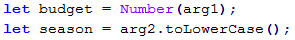Always take into consideration what value type is passed in the input data, as well as what type these need to be converted to, for the program conditions to work properly!

#### Calculations

Let's create and initialize the variables needed for applying the logic and calculations: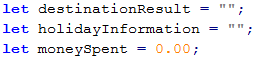Similar to the example in the previous task, we can initialize variables with some of the output results, to spare additional initialization.

When examining once again the problem requirements, we notice that the main distribution of where the vacation will take place is determined by the value of the budget, i.e. our main logic is divided into two cases:

• If the budget is less than a particular value.
• If it is less than another value or is more than the specified border value.

Based on the way we arrange the logical scheme (the order in which we will check the border values), we will have more or fewer conditions in the solution. Think why!

After that, we need to apply a condition to check the value of the season. Based on it, we will determine what percentage of the budget will be spent, as well as where the programmer will stay – in a hotel or a camp.

This is a sample code that may be used to implement the above idea:We can optimize the conditions checking by assigning a default value and then checking one variant less. This saves one logical step.

For example, this block: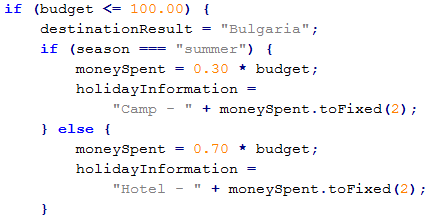can be shortened like this:#### Printing The Result

What remains is to display the calculated result on the console:### Testing in The Judge System

Test your solution here: https://judge.softuni.org/Contests/Practice/Index/932#1.

## Problem: Operations

Write a program that reads two integers (n1 and n2) and an operator that performs a particular mathematical operation with them. Possible operations are: summing up (+), subtraction (-), multiplying (*), division (/) and modular division (%). Upon summing up, subtracting, and multiplying, the console must print the result and display whether it is an even or an odd number. Upon regular division – just the result, and upon modular division – the remainder. You need to take into consideration the fact that the divisor can be equal to zero (= 0) and dividing by zero is not possible. In this case, a special notification must be printed.

### Input Data

3 arguments are passed to the function:

• N1integer within the range [0 … 40 000].
• N2integer within the range [0 … 40 000].
• Operatorone character among: "+", "-", "*", "/", "%".

### Output Data

Print the output as a single line on the console:

• If the operation is summing up, subtraction or multiplying:
• "{N1} {operator} {N2} = {output} – {even/odd}".
• If the operation is division:
• "{N1} / {N2} = {output}" – the result is formatted up to the second digit after the decimal point.
• If the operation is modular division:
• "{N1} % {N2} = {remainder}".
• In case of dividing by 0 (zero):
• "Cannot divide {N1} by zero".

### Sample Input and Output

Input Output Input Output
123
12
/
123 / 12 = 10.25 112
0
/
Cannot divide 112 by zero
10
3
%
10 % 3 = 1 10
0
%
Cannot divide 10 by zero
Input Output
10
12
+
10 + 12 = 22 - even
10
1
-
10 - 1 = 9 - odd
7
3
*
7 * 3 = 21 - odd

### Hints and Guidelines

The problem is not complex, but there are a lot of code lines to write.

#### Processing The Input Data

Upon reading the requirements, we understand that we expect three parameters of input data. The first two parameters are integers (within the specified range), and the third one – an arithmetical symbol.#### Calculations

Let's create and initialize the variables needed for the logic and calculations. In one variable we will store the calculations output, and in the other one, we will use it for the final output of the program.When carefully reading the requirements, we understand that there are cases where we don't need to do any calculations, and simply display a result.

Therefore, we can first check if the second number is 0 (zero), as well as whether the operation is a division or a modular division, and then initialize the output.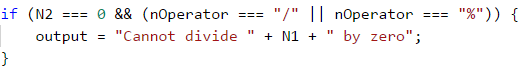Let's place the output as a value upon initializing the output parameter. This way we can apply only one condition – whether it is needed to recalculate or replace this output.

Based on the approach that we choose, our next condition will be either a simple else or a single if. In the body of this condition, using additional conditions regarding the manner of calculating the output based on the passed operator, we can separate the logic based on the structure of the expected output.

From the requirements we can see that for summing up (+), subtraction (-) or multiplying (*) the expected output has the same structure: "{n1} {operator} {n2} = {output} – {even/odd}", whereas for division (/) and modular division (%) the output has a different structure.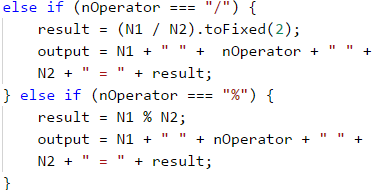We finish the solution by applying conditions for summing up, subtraction, and multiplying: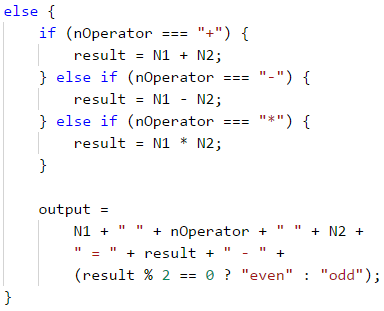For short and clear conditions, such as the above example for even and odd numbers, you can use a ternary operator. Let's examine the possibility to apply a condition with or without a ternary operator.

Without using a ternary operator the code is longer but easier to read: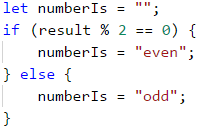Upon using a ternary operator the code is much shorter, but may require additional efforts to read and understand the logic:#### Printing The Output

Finally, what remains is to print the calculated result on the console:### Testing in The Judge System

Test your solution here: https://judge.softuni.org/Contests/Practice/Index/932#2.

## Problem: Match Tickets

A group of football fans decided to buy tickets for Euro Cup 2016. The tickets are sold in two price categories:

• VIP499.99 BGN (Bulgarian leva).
• NormalNormal249.99 BGN (Bulgarian leva).

The football fans have a shared budget, and the number of people in the group determines what percentage of the budget will be spent on transportation:

• 1 to 4 – 75% of the budget
• 5 to 9 – 60% of the budget
• 10 to 24 – 50% of the budget
• 25 to 49 – 40% of the budget
• 50 or more – 25% of the budget

Write a program that calculates whether the money left in the budget will be enough for the football fans to buy tickets in the selected category, as well as how much money they will have left or be insufficient.

### Input Data

The input data contains exactly 3 lines (arguments):

• The first line (argument) contains the budget – a real number within the range [1 000.00 … 1 000 000.00].
• The second line (argument) contains the category – "VIP" or "Normal".
• The third line (argument) contains the number of people in the group – an integer within the range [1 … 200].

### Output Data

Print the following on the console as one line:

• If the budget is sufficient:
• "Yes! You have {N} leva left." – where N is the amount of remaining money for the group.
• If the budget is NOT sufficient:
• "Not enough money! You need {M} leva." – where M is the insufficient amount.

The amounts must be formatted up to the second digit after the decimal point.

### Sample Input and Output

Input Output Explanations
1000
Normal
1
Yes! You have 0.01 leva left. 1 person: 75% of the budget is spent on transportation.
Remaining amount: 1000 – 750 = 250.
Category Normal: the ticket price is 249.99 * 1 = 249.99
249.99 < 250: the person will have 250 – 249.99 = 0.01 money left
Input Output Explanations
30000
VIP
49
Not enough money! You need 6499.51 leva. 49 people: 40% of the budget are spent on transportation.
Remaining amount: 30000 - 12000 = 18000.
Category VIP: the ticket costs 499.99 * 49.
24499.510000000002 < 18000.
The amount is not enough 24499.51 - 18000 = 6499.51

### Hints and Guidelines

We will read the input data and perform the calculations described in the task requirements, to check if the money will be sufficient.

#### Processing The Input Data

Let's read carefully the requirements and examine what we expect to take as input data, what is expected to return as a result, as well as what are the main steps for solving the problem.

For a start, let's process and save the input data in appropriate variables:#### Calculations

Let's create and initialize the variables needed for doing the calculations: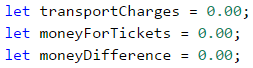Let's review the requirements once again. We need to perform two different block calculations.

By the first set of calculations, we must understand what part of the budget has to be spent on transportation. You will notice that the logic for doing these calculations only depends on the number of people in the group. Therefore, we will do a logical breakdown according to the number of football fans.

We will use a conditional statement – a sequence of if-else blocks.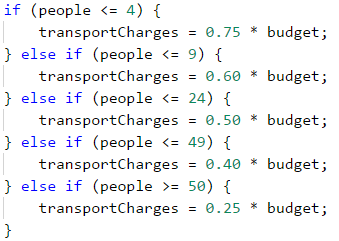By the second set of calculations, we need to find out what amount will be needed for purchasing tickets for the group. According to the requirements, this only depends on the type of tickets that we need to buy.

Let's use a switch-case conditional statement.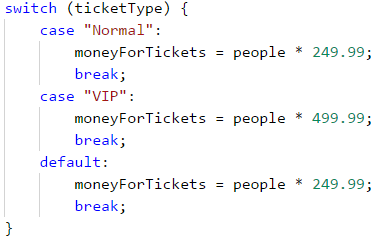Once we have calculated the transportation costs and ticket costs, what remains is to calculate the final result and understand if the group of football fans will attend Euro Cup 2016 or not, by the provided the available parameters.

For the output, to spare one else condition in the construction, we will assume that the group can, by default, attend Euro Cup 2016.#### Printing The Result

Finally, we need to display the calculated result on the console.

### Testing in The Judge System

Test your solution here: https://judge.softuni.org/Contests/Practice/Index/932#3.

## Problem: Hotel Room

A hotel offers two types of rooms: studio and apartment.

Write a program that calculates the price of the whole stay for a studio and apartment. Prices depend on the month of the stay:

May and October June and September July and August
Studio – 50 BGN/per night Studio – 75.20 BGN/per night Studio – 76 BGN/per night
Apartment – 65 BGN/per night Apartment – 68.70 BGN/per night Apartment – 77 BGN/per night

The following discounts are also offered:

• For a studio, in case of more than 7 stays in May and October: 5% discount.
• For a studio, in case of more than 14 stays in May and October: 30% discount.
• For a studio, in case of more than 14 stays in June and September: 20% discount.
• For an apartment, in case of more than 14 stays, no limitation regarding the month: 10% discount.

### Input Data

The input data contains exactly two lines (arguments):

• The first (argument) line contains the monthMay, June, July, August, September or October.
• The second line (argument) is the number of stays – integer within the range [0 … 200].

### Output Data

In this problem, our currency will be lv, which is BGN (Bulgarian lev). Print the following two lines on the console:

• On the first line: "Apartment: { price for the whole stay } lv."
• On the second line: "Studio: { price for the whole stay } lv."

The price for the whole stay must be formatted up to two symbols after the decimal point.

### Sample Input and Output

May
15
Apartment: 877.50 lv.
Studio: 525.00 lv.
In May, in case of more than 14 stays, the discount for a studio is 30% (50 - 15 = 35), and for the apartment is 10% (65 - 6.5 = 58.5).
The whole stay in the apartment: 877.50 lv.
The whole stay in the studio: 525.00 lv.
Input Output
June
14
Apartment: 961.80 lv.
Studio: 1052.80 lv
August
20
Apartment: 1386.00 lv.
Studio: 1520.00 lv.

### Hints and Guidelines

We will read the input data and do the calculations according to the provided price list and the discount rules, and finally, print the result.

#### Processing The Input Data

According to the task requirements, we expect two parameters, that contain the input data - the first parameter is the month in which the stay is planned, and the second - the number of stays.

Let's process and store the input data in the appropriate parameters: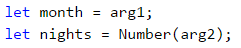#### Calculations

Now let's create and initialize the variables needed for the calculations: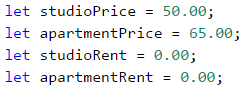When doing an additional analysis of the requirements, we understand that our main logic depends on what month is passed and what is the number of stays.

In general, there are different approaches and ways to apply the above conditions, but let's examine a basic switch-case conditional statement, as in the individual case blocks we will use if and if-else conditional statements.

Let's start with the first group of months: May and October. For these two months, the price for a stay is the same for both types of accommodation – a studio or an apartment. Therefore, the only thing that remains is to apply an internal condition regarding the number of stays and recalculate the relevant price (if needed):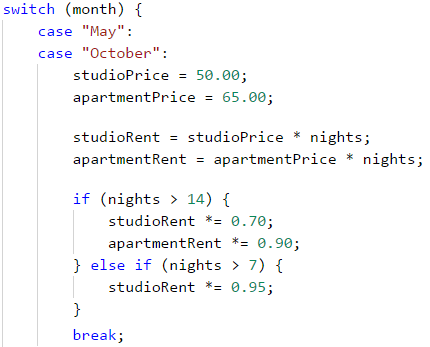To some extent, the logic and calculations will be identical for the following months: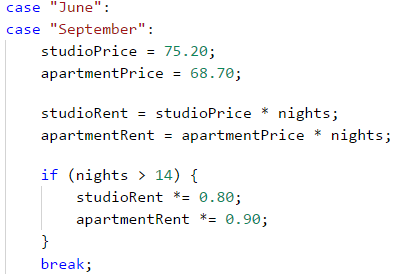After calculating the relevant prices and the total amount for the stay, now let's prepare the formatted result. Before that, we should store it in our output parametersstudioInfo and apartmentInfo:To calculate the output parameters, we will use the .toFixed(Number) method. This method rounds the decimal number up to a specified number of characters after the decimal point. In our case, we will round the decimal number up to 2 digits after the decimal point.

#### Printing The Result

Finally, what remains is to print the calculated results on the console.

### Testing in The Judge System

Test your solution here: https://judge.softuni.org/Contests/Practice/Index/932#4.